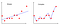# Most Underrated component of ML : Regularization

Hello Guys, This blog contains all you need to know about regularization. This blog is all about mathematical intuition behind regularization and its implementation in python.This blog is intended specially for newbies who are finding regularization difficult to digest. For any machine learning enthusiast , understanding the mathematical intuition and background working is more important then just implementing the model.

I came across many blogs which were answering why regularization is needed and what it does but failed to answer how?

What is Overfitting?

Each dataset comprises of some amount of noise. When your model tries to fit your data too well then you crash into over fitting. Overfitting tries to reach every noise data thus increases complexity. The repercussions of overfitting is that your model’s training accuracy will be too high whereas testing accuracy will be too low. This means that your model will fail to predict new/real-time data.Right Image : Over-fitting Data

One way of avoiding over-fitting is to penalise the weights/coefficients and this is exactly what regularization does.

# Regularization

It is a form of regression, that constrains or shrinks the coefficient estimating towards zero. In other words, this technique discourages learning a more complex or flexible model, so as to avoid the risk of over-fitting.

There are mainly two types of regularization:

1. Ridge Regression

I’ll explain generalised mathematical intuition by taking ridge regression into context which would be further same for other types except the regularization term. Imagine you’re working on simple linear regression.

So, the formula for hypothesis would be:

Loss Function be:

Here, J(theta)/Loss is the loss function for a model. It is different from normal loss function as regularization term is added to penalise the coefficients in the end. Lambda is regularization rate.

Here, Regularization term is lambda multiplied to the summation of square of coefficients. Therefore adding regularization term, increases cost function as compared to normal cost function

Note: Normal cost function is the same as shown in above figure but doesn’t have regularization term So don’t get confuse.

In each model after computing cost we use any optimising algorithm to improve our weights. In this case we’ll use gradient descent for optimisation.

Gradient Descent is a process to find optimal weights/coefficient which is turn used to predict new samples/data.

In Gradient Descent, new weights are obtained when learning rate (alpha) is multiplied with derivative(differentiation) of cost function and deducted from old weight. This process occurs for multiple iterations where weights are optimised simultaneously.

As we saw earlier that adding regularization term increases cost function so when derivative of cost function is deducted from weights in gradient descent then new weights would be much more lesser as compared to that we obtained using normal cost function(without regularization).

In simple words, if cost function without regularization is termed as X and cost function with regularization is termed as Y then it is obvious that Y>X as regularization term is added. Also new weights computed using cost function with regularization is W1 and new weights computed using cost function without regularization is W2 then W2>W1.

The above two figures shows how gradient descent works in detail. It takes Simple linear Regression as model under consideration.

Coming back to Ridge Regression, Drawback of ridge regressor is that it can reduce the coefficients/weights to minimum threshold but can’t reduce it to zero. Ridge regression is used when multi-collinearity occurs. Multi-collinearity means features are dependent on each other.

2. Lasso Regression (L1 Regression)

Lasso follows same mathematical intuition as stated above in ridge regression. The only difference is in regularization term. Regularization term is regularization rate multiplied with summation of modulus of weights. Lasso Regression overcomes the drawback of ridge regression by not only penalising the coefficients but also setting them to zero if not relevant to dependent variable.

So, Lasso regression can also be used as feature selection and it comes under embedded methods of feature selection.

Implementation

`from sklearn.linear_model import Lassomodel = Lasso(alpha=0.6)model.fit(Xtrain, ytrain)ypred = model.predict(Xtest, ytest)`

Similarly, sklearn library provides functionality for Ridge. It also expands to RidgeCV and LassoCV.

How to use Regularization Rate ?

On implementing any of these regression techniques If you are facing :

(1) Overfitting → Try to increase the regularization rate.

(2) Underfitting → Try to decrease the regularization rate.

End Notes

In this article we got a general understanding of regularization. In reality the concept is much deeper than this.

Did you find the article useful? Do let us know your thoughts about this article in the comment box below.

That’s all folks, Have a nice day :)

2X Kaggle Expert | Jr. Machine Learning Developer at Infomize Technologies

## More from Dhruv Gangwani

2X Kaggle Expert | Jr. Machine Learning Developer at Infomize Technologies